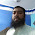### XI Accounting Class 09 Accounting Equation Problems

XI Accounting Class 09 Accounting Equation Problem #01

ACCOUNTING EQUATION
Assets = liabilities + owner’s equity
liabilities = Assets - owners equity
owner’s equity = Assets - liabilities

Question # 01
Total assets 300,000 and total liabilities 150,000 calculate Equity?
Solution
Accounting Equation
Assets = liabilities + owner’s equity

Owner’s equity = Assets - liabilities
Owner’s equity = 300,000-150,000

 Owner’s equity = 150,000

Question # 02
total liabilities 150,000 and owner equity 150,000 calculate assets?
Solution
Accounting Equation
Assets = liabilities + owner’s equity

Assets = 150,000    + 150,000

 Assets = 300,000

Question # 03
Total assets 110,000 and equity 90,000 calculate liabilities?
Solution
Accounting Equation
Assets = liabilities + owner’s equity

Liabilities = Assets - owner’s equity
Liabilities = 110,000 - 90,000

 Liabilities = 20,000

Question # 03 (a) The assets of business entity having liabilities of Rs.60,000 and owner’s equity Rs.120,000

Solution
Accounting Equation
Assets = liabilities + owner’s equity

Assets =  60,000 +  120,000

 Assets = 180,000

b. The owner’s equity of business entity having assets of Rs.600,000 and liabilities
Rs.250,000.

Solution
Accounting Equation
Assets = liabilities + owner’s equity

Owner’s equity =  Assets - liabilities
Owner’s equity =  600,000 - 250,000

 Owner’s equity =  350,000

c.  The liabilities of business entity having assets of Rs.310,000 and owner’s equity
Rs.70,000.
Solution
Accounting Equation
Assets = liabilities + owner’s equity

Liabilities = Assets - owner’s equity
Liabilities =  310,000 - 70,000

 Liabilities = 240,000

Question # 4)1993 Regular & Private – BIEK For each of the following determine the underlined missing item:-

(a) The liabilities of a business entity having assets of Rs.200,000 and owner’s equity of
Rs.90,000.
Solution

Accounting Equation
Assets = liabilities + owner’s equity

Liabilities = Assets - owner’s equity

Liabilities =  200,000 - 90,000

 Liabilities = 110,000

(b) The assets of a business entity having liabilities of Rs.50,000 and owner’s equity of
Rs.100,000.

Solution

Accounting Equation
Assets = liabilities + owner’s equity

Assets =  50,000 +  100,000

 Assets = 150,000

(c) The owner’s equity of a business having assets of Rs.80,000 and liabilities of Rs.40,000.
Solution

Accounting Equation
Assets = liabilities + owner’s equity

Owner’s equity = Assets - liabilities

Owner’s equity =  80,000 - 40,000

 Owner’s equity = 40,000

(d) The revenue of a business entity having expenses of Rs.60,000 and net income of
Rs.15,000
Solution
Revenue = expense + Profit/net income
.Revenue = 60,000  + 15,000

 Revenue =  75,000
.

(e) The expenses of a business entity having revenues of Rs.90,000 and net loss of Rs.8,000.

Solution

Expense = Revenue + Loss

Expense =  90,000 + 8,000

 Expense =  98,000
.

Question # 5)  2002 Private – BIEK  For each of the following determine the underlined missing item:-
(a) The liabilities of a business entity having assets of Rs.400,000 and owner’s equity of
Rs.180,000.
Solution
Accounting Equation
Assets = liabilities + owner’s equity

Liabilities = Assets - owner’s equity
Liabilities =  400,000 - 180,000

 Liabilities = 220,000
(b) The assets of business entity having liabilities of Rs.100,000 and owner’s equity of
Rs.200,000.
Solution
Accounting Equation
Assets = liabilities +   owner’s equity
Assets = 100,000    + 200,000

 Assets = 300,000

(c) The owner’s equity of business having assets of Rs.1600,000 and liabilities of Rs.80,000.
Solution

Accounting Equation
Assets = liabilities + owner’s equity

Owner’s equity = Assets - liabilities

Owner’s equity =  1600,000 - 80,000

 Owner’s equity = 1,520,000

(d) The revenues of business entity having expenses of Rs.120,000 and net income of Rs.30,000.
Solution
Revenue = expense + Profit/net income
Revenue = 120,000  + 30,000

 Revenue =  150,000

(e) The expenses of a business entity having revenues of Rs.180,000 and net loss of Rs.16,000.

Solution
Expense = Revenue + Loss
Expense =  180,000 + 16,000

 Expense =  196,000

Question # 6:
1994 Regular & Private – BIEK  Compute the missing amount in each of the following independent cases:-

(i)   The assets of Javeed Company total Rs.350,000 and the owner’s equity amounts to
Rs.110,000. What is the amount of liabilities?

Solution

Accounting Equation
Assets = liabilities + owner’s equity

Liabilities = Assets - owner’s equity

Liabilities =  350,000 - 110,000

 Liabilities = 240,000

(ii)   The balance sheet of Star Company shows owner’s equity of Rs.80,000 which is equal
to one third amount of total assets. What is the amount of liabilities?

Solution

Compute one third amount of total assets.
80,000x3/1

Assets = 240,000

Accounting Equation
Assets = liabilities + owner’s equity

Liabilities = Assets - owner’s equity
Liabilities =  240,000 - 80,000

 Liabilities = 160,000

(iii) Moon Company had assets in the amount of Rs.230,000 on December 31, 1988. Assets
increased to Rs.320,000 by December 31 of 1989. During this same period, liabilities
increased by Rs.85,000. The owner’s equity at December 31, 1988 amounted to
Rs.160,000. Compute the amount of owner’s equity at December 31, 1989.
Solution
Accounting Equation
Assets = liabilities + owner’s equity
1988
Liabilities = Assets - owner’s equity
Liabilities =  230,000 - 160,000

 Liabilities = 70,000

Owner's Equity And Income or Revenue

List Of Expenses

Complete List of Heads Of Accounts & Normal Balance

Accounting Equation

Accounting Equation
Assets = liabilities + owner’s equity
1989
owner’s equity = Assets -  Liabilities
owner’s equity =  320,000 - (70,000 + 85,000)

owner’s equity = 320,000 - 155,000
owner’s equity =  165,000

1.Sir, How to solve this question.
The balance sheet of a company shows liabilities of Rs. 50,000 which is equal to one- fourth the amount of total assets.

1.dear student, it is really simple
one forth mean 1/4
first step we have to find the amount of total assets
50,000x1/4= 12500
formula
owner’s equity = Assets - Liabilitie
37500 = 12500 - 50,0000

If You Face Any Problem feel free to ask
ager kio masla ho to app yaha pouch saktey hai